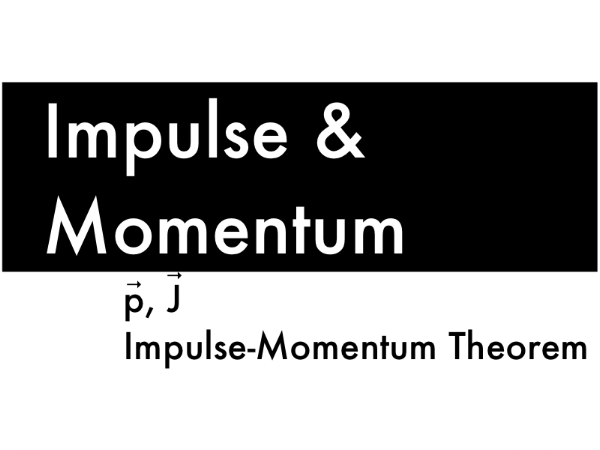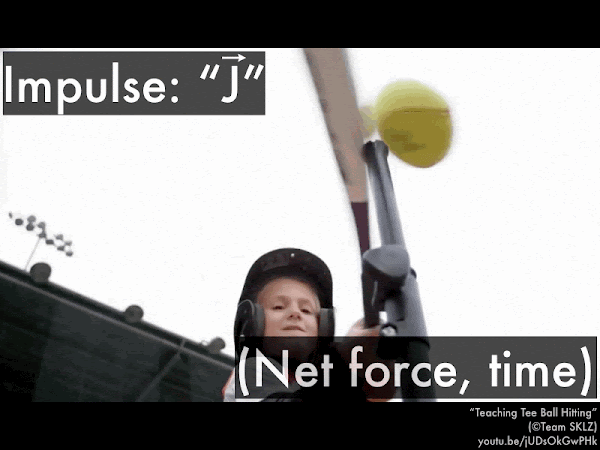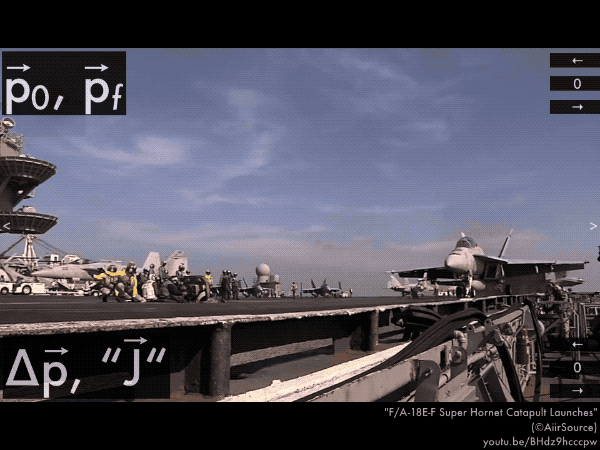## 20170929

### Physics presentation: impulse and momentumWhuuuuuuut. (Video link: "bowling strike with a ping pong ball.")In this presentation we will introduce another new connection between forces and motion, in terms of how the net force can exert an impulse on an object in order to change its momentum. This is yet another new approach to connecting forces and motion, compared to the previous discussion in this course of using Newton's laws to relate how forces on an object result in a net force that may or many not change its motion, and analyzing how forces can do work on or against an object in order to speed up or slow down its motion.First, defining the momentum of an object, and then expressing how the net force can exert an impulse on this object.The introduction slide showing a ping-pong ball knocking over all ten bowling pins should seem very strange to you, as the mass of the ping-pong ball is too small to effectively bowl a strike, even if it were traveling with a supersonic speed. In order to fully account for the "knocking-over" strength of a moving object, then, we must include mass as well as its speed (and direction) to define its momentum p.Momentum p is a vector quantity (so don't forget to draw an arrow over it) whose magnitude depends both on the mass and speed of the object, with the combined units of both mass and speed (kg·m/s).We also need to introduce the concept of impulse J, which is the product of the net force acting on an object and the duration of time that the net force acted on this object (whether for a brief instant, or for a prolonged period). (Video link: "Teaching Tee Ball Hitting.")Impulse has the combined units of both force and time (N·s). Here we use the somewhat obscure (but totally legit) "J" symbol for impulse, remembering to draw an arrow over it (as it is a vector quantity). (It turns out that "I" is already reserved for rotational inertia in the next chapter.)Second, let's now explicitly make the connection between the impulse acting on an object, and the resulting change in the momentum of the object.This "impulse-momentum theorem" emphasizes how the impulse (exerted by the net force acting over a specific duration of time) causes a corresponding initial-to-final change in the momentum of the object. And vice versa, where the initial-to-final change in the momentum of an object is caused by the impulse on the object.Let's apply these concepts to several objects that undergo changes in momentum, with an emphasis on the directions (+/– signs) of these quantities, and how they all must be consistent with each other, starting with a golf ball initially at rest, and then has a speed of 97 m/s after being hit by a golf club. (Video link: "The Moment of Impact. An Inside Look at Titleist Golf Ball R&D.")

This golf ball is initially at rest, so its initial momentum p0 (mass times its initial velocity) is 0.

We'll define the horizontal direction to be positive to the right (and negative to the left). After it is hit by the golf club, its final momentum (mass times its final velocity) pf points to the right (and will be a positive quantity).

The initial-to-final change in momentum ∆p of the golf ball is given by:

p = pfp0,

and since get a positive quantity minus zero, then ∆p must be positive (thus pointing to the right).

Since the impulse "J" on the golf ball causes this initial-to-final change in momentum:

"J" = ∆p,

the impulse must also have the same direction as ∆p, and so it must also point to the right. (Also since the impulse "J" is the net force ΣF on the golf ball times the contact time ∆t, the net force of the golf club on the golf ball is also directed to the right.)Now let's have you look at the directions involved in the impulse-momentum theorem for this catapult-launched F/A-18E-F Super Hornet, initially at rest, and then has a speed of 74 m/s after being it is catapulted. (Video link: "F/A-18E-F Super Hornet Catapult Launches.")

Super Hornet's initial momentum p0 direction? (left (–), none (0), or right (+)?)
Super Hornet's final momentum pf direction?
Direction of Super Hornet's initial-to-final change in momentum ∆p?
Direction of catapult's impulse "J" on the Super Hornet?Finally, consider the directions involved in the impulse-momentum theorem for this Ford Ranger, hitting a crash barrier with a speed of 11.0 m/s, and then rebounding off the crash barrier with a speed of 2.2 m/s. (Video link: "Crash Test Ford Ranger 2012....")
Ford Ranger's initial momentum p0 direction? (left (–), none (0), or right (+)?)
Ford Ranger's final momentum pf direction?
Direction of Ford Ranger's initial-to-final change in momentum ∆p?